# OECD Crop production data analysis 5 - Using pivot_wider(), select() and rename() function to make a new "wider format" data frame.This post is followiing of the above post.

In this post, I will make wider format data frame from "df" data frame object.

First, let's see "df" data frame.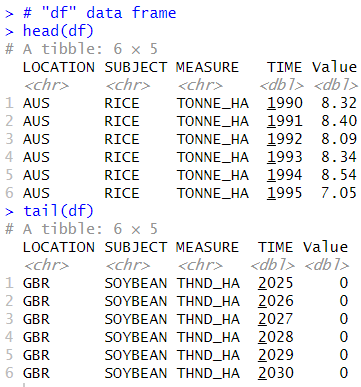I see SUBJECT variable has 4 kinds of values, MAIZE, RICE, WHEAT and CORN, MEASURE variable has 3 koids of values, TONNE_HA, THND_HA and THND_TONNE.

So, actually, this data has 4 * 3 = 12 kinds of values.

I will make a data frame which have those each 12 variables.

I use pivot_wider() function.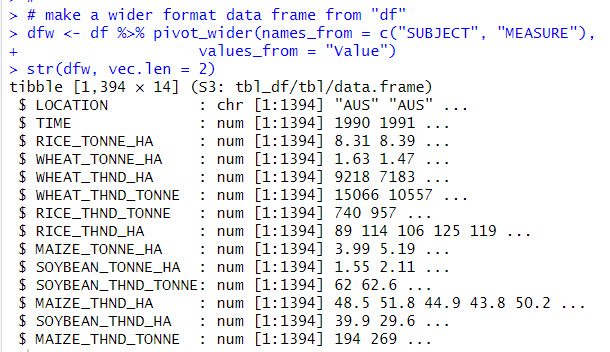Now, I made a new data frame named "dfw" which has LOCATION, TIME and those 12 variables.

I use rename function to rename each variables to more simple names.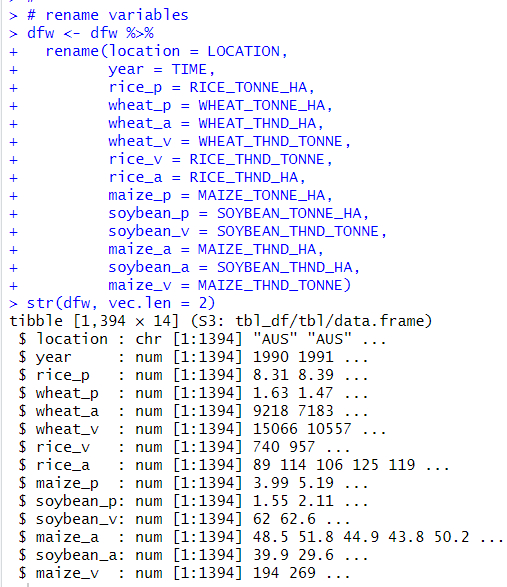"_p" means productivity, TONNE_HA.

"_a" means field area, THND_HA.

"_v" means crop volume, THND_TONNE.

Next, I use select() to reorder variables.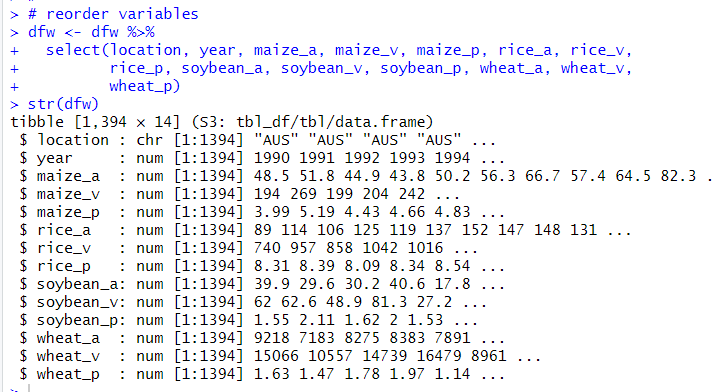Next, I converted location class to factor from character using as.factor() function.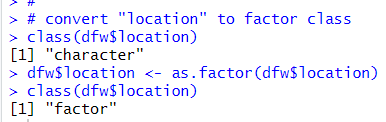All right, now I completed converting long format data frame "df" to wide format data frame "dfw".

Let's use summary() function to see basic statistics.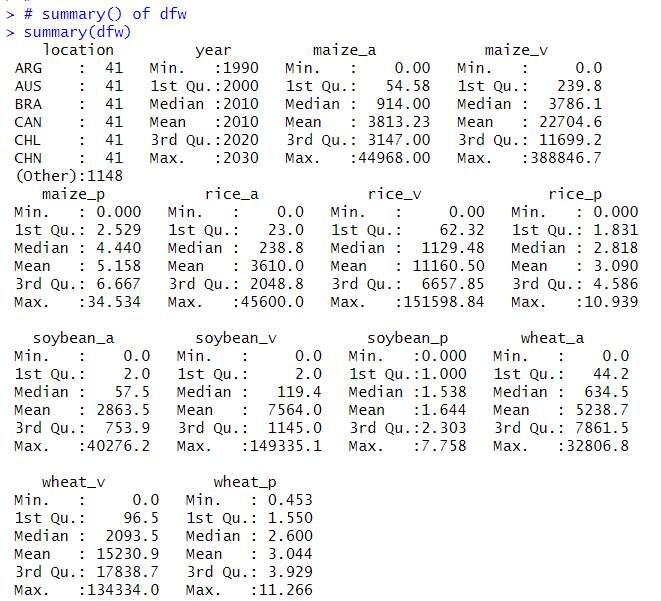I see each variables have 0 value.

Wheat has the largest crop field. Maize has the largest crop volume, Maize has the highest productivity.

That's it. Thank you!

Next post is

To read from the first post,